Cycle decompositionNext: Conjugation Up: Actions Previous: Finite symmetric groups

# Cycle decomposition

The points which form the first row need not be written in their natural order, e.g.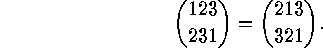Keeping this in mind, we call a permutation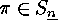a cyclic permutation or a cycle   if and only if it can be written in the form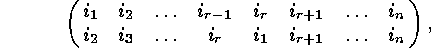where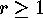. In order to emphasize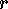we also call it an r-cycle  . We note that in this case the orbits of the subgroup generated by this permutation are the following subsets of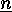: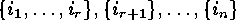. We therefore abbreviate this cycle by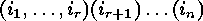, where the points which are cyclically permuted are put together in round brackets. For example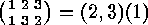. Commas which seperate the points may be omitted if no confusion can arise (e.g if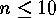), and 1-cycles can be left out if it is clear which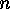is meant. Hence we can write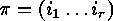for the-cycle introduced above. This cycle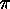can also be expressed in terms of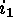alone: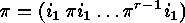. Using all these abbreviations and denoting by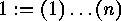the identity element, we obtain for example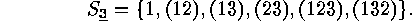There are the elements of the symmetric group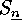in cycle notation. The notation for a cyclic permutation is not uniquely determined, since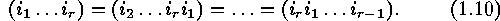2-cycles are called transpositions  . The order of a cycle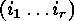, i.e. the order of the cyclic group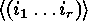generated by this cycle, is equal to its length :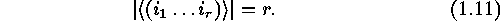Two cyclesand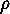are called disjoint  , if the two sets of points which are not fixed byandare disjoint sets. Notice that, for example,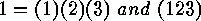are disjoint cycles. Disjoint cyclesandcommute, i.e.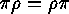. (We read compositions of mappings from right to left, so that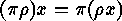.) Each permutation of a finite set can be written as a product of pairwise different disjoint cycles, e.g.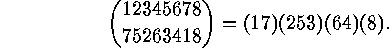There are some more examples for the cycle decomposition of a permutation.

The disjoint cyclic factors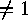ofare uniquely determined byand therefore we call these factors together with the fixed point cycles ofthe cyclic factors of. Let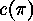denote the number of these cyclic factors of(including 1-cycles), let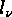be their lengths,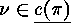(recall that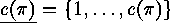), choose, for each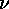an element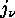of the-th cyclic factor. Then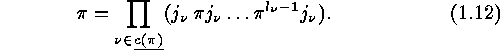This notation becomes uniquely determined if we choose theso that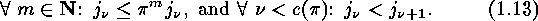If this holds, thenis called the (standard) cycle notation for. We note in passing that the sets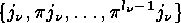of points which are cyclically permuted byare just the orbits of the cyclic group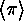generated by.Next: Conjugation Up: Actions Previous: Finite symmetric groups

Herr Fripertinger
Sun Feb 05 18:28:26 MET 1995